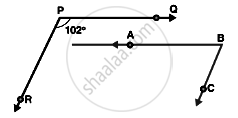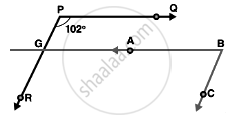# In the Below , Pq || Ab and Pr || Bc. If ∠ Qpr = 102°, Determine ∠ Abc. Give Reasons. - Mathematics

Sum

In the below, PQ || AB and PR || BC. If ∠QPR = 102°, determine ∠ABC. Give reasons.#### Solution

AB is produce to meet PR at K

Since PQ || AB

∠QPR = ∠BGR = 102°  ...[corresponding angles]

Since PR || BC

∴∠RGB + ∠CBG = 180°        ...[Corresponding angles are supplementary]

⇒ ∠CBG =180° - 102° = 78°

∴ ∠CBG = ∠ ABC

∠ ABC = 78°.Concept: Pairs of Angles
Is there an error in this question or solution?
Chapter 10: Lines and Angles - Exercise 10.4 [Page 49]

#### APPEARS IN

RD Sharma Mathematics for Class 9
Chapter 10 Lines and Angles
Exercise 10.4 | Q 22 | Page 49

Share